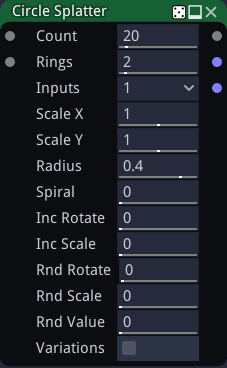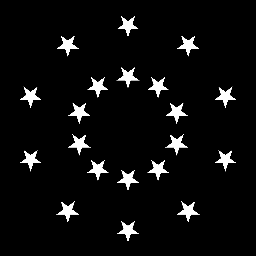# Circle Splatter node¶

The Circle Splatter node splats several instances of its input around a circle or a spiral. Overlapping instances are mixed with each other using a lighten filter.

The Circle Splatter also has a color version whose input is in RGBA format.## Inputs¶

The Circle Splatter node accepts two inputs:

• The Source inputs is the image to be splat into the output.

• The Mask input is a greyscale image that is used as a mask and affects each instance’s value.

## Outputs¶

The Circle Splatter node outputs the splat image.

The greyscale circle splatter has two additional outputs, one that assigns a random color to each splat instance and one that assigns a UV layout to each splat instance.

## Parameters¶

The Circle Splatter node accepts the following parameters:

• Count, the number of instances of the source image in the result, including those canceled by the mask.

• Rings, the number of rings of the circle or spiral pattern.

• Inputs is the number of alternate shapes in the input (1, 4 or 16). Images containing several shapes can easily be created using the Tile2x2 node.

• Scale X and Scale Y are the scale along X and Y axes applied to each instance.

• Radius is the radius of the outer circle (or distance to center of the outer instance when a spiral is generated)

• Spiral can be used to select between a circle or a spiral pattern (or anything in between)

• IncRotate is the rotation increment applied to each instance.

• IncScale is the scale increment applied to each instance.

• RndRotate is the maximum angle of the random rotation applied to each instance.

• RndScale is the amount of random scaling applied to each instance.

• RndValue is the amount of random value applied to each instance.

• Variations: if checked, the node will tile different variations of its input (i.e. roll a different seed for each instance)

## Example images¶